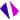# 預備知識章節介紹課程內容
1.1 function_01
1.2 Equations of lines
1.3 inverse function
1.4 exponential and logarithmic functions
1.5 Trigonometric and inverse trigonometric functions
1.6 hyperbolic and inverse hyperbolic function
 課程講義 課程影音1.1 節講義1.2 節講義1.3 節講義1.4 節講義1.5 節講義1.6 節講義1.1_function_011.1_function_021.2 Equations of lines1.3 inverse function1.4 exponential and logarithmic functions1.6 hyperbolic and inverse hyperbolic function